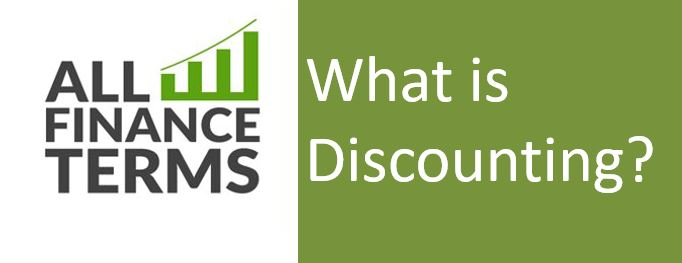## Definition of Discounting

Discounting means calculating the present value PV of amount which is expected to be received at some time in future. Time value of money is the concept behind discounting.

### Brief Explanation Of  Discounting

Receiving \$ 1M would worth more if received now than after three years because receiving it today you can utilize it like investing and gaining interest than receiving it after three years. Hence the process of finding present value or current value of the future cash inflow of money using a reasonable rate of interest (also known as discounting rate) is called discounting.

This technique also helps businesses to decide whether to lend their money considering the future inflation and expected rate of return.

Formula:

The factors involved in calculating discounted rates are;

• N= Time period/years involved
• PV or DCF (Discounted Cash flow) = Present value
• FV=Future Value
• R=Annual rate of return

PV / DCF =FV *(1+R) ^(-N) or FV *(1+R)/(N)

For Example:

Suppose we are to receive \$ 1000 after two years. Now how to calculate its present value assuming Discounting rate is 10 % is mentioned below;

FV which would be received = \$ 1000

N = 2

R = 10 %

Putting in formula

PV =    \$ 1000* (1+10%) ^ (-2)

PV =    \$ 1000 * (1.10) ^ (-2)

PV =   \$ 1000/ 0.826446

PV =    \$ 826.4

This answer \$ 826.4, shows us that receiving \$1000 in two years is the same as receiving \$ 826.4 today, if the time value of money is 10 % per year.

Using same example with different discounting rate, say 20 % let us see the results

PV =    \$ 1000* (1+20%) ^ (-2)

PV =    \$ 1000 * (1.20) ^ (-2)

PV =   \$ 1000/ 0.695

PV =    \$ 695

This shows us that the higher the discount rate, the lower the present value of future cash flows.